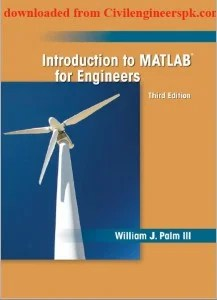# Introduction to MATLAB for Engineers

By William J. Palm III,  Third Edition### Contents of Introduction to MATLAB for Engineers

Chapter One
1.1–1 Volume of a circular cylinder
1.6–1 Piston motion
Chapter Two
2.3–1 Vectors and displacement
2.3–2 Aortic pressure model
2.3–3 Transportation route analysis
2.3–4 Current and power dissipation in resistors
2.3–5 A batch distillation process
2.4–1 Miles traveled
2.4–2 Height versus velocity
2.4–3 Manufacturing cost analysis
2.4–4 Product cost analysis
2.5–1 Earthquake-resistant building design
2.6–1 An environmental database
2.7–1 A student database
Chapter Three
3.2–1 Optimization of an irrigation channel
Chapter Four Introduction to MATLAB for Engineers
4.3–1 Height and speed of a projectile
4.5–1 Series calculation with a for loop
4.5–2 Plotting with a for loop
4.5–3 Data sorting
4.5–4 Flight of an instrumented rocket
4.6–1 Series calculation with a while loop
4.6–2 Growth of a bank account
4.6–3 Time to reach a speci ed height
4.7–1 Using the switch structure for calendar calculations
4.9–1 A college enrollment model: Part I
4.9–2 A college enrollment model: Part II
Chapter Five Introduction to MATLAB for Engineers
5.2–1 Plotting orbits
Chapter Six Introduction to MATLAB for Engineers
6.1–1 Temperature dynamics
6.1–2 Hydraulic resistance
6.2–1 Estimation of traf c ow
6.2–2 Modeling bacteria growth
6.2–3 Breaking strength and alloy
composition
6.2–4 Response of a biomedical instrument
Chapter Seven Introduction to MATLAB for Engineers
7.2–1 Mean and standard deviation of heights
7.2–2 Estimation of height distribution
7.3–1 Statistical analysis and manufacturing tolerances
Chapter Eight Introduction to MATLAB for Engineers
8.1–1 The matrix inverse method
8.2–1 Left division method with three unknowns
8.2–2 Calculations of cable tension
8.2–3 An electric resistance network
8.2–4 Ethanol production
8.3–1 An underdetermined set with three equations and three unknowns
8.3–2 A statically indeterminate problem
8.3–3 Three equations in three unknowns, continued
8.3–4 Production planning
8.3–5 Traf c engineering
8.4–1 The least-squares method
8.4–2 An overdetermined set
Chapter Nine Introduction to MATLAB for Engineers
9.1–1 Velocity from an accelerometer
9.1–2 Evaluation of Fresnels cosine integral
9.1–3 Double integral over a nonrectangular region
9.3–1 Response of an RC circuit
9.3–2 Liquid height in a spherical tank
9.4–1 A nonlinear pendulum model
9.5–1 Trapezoidal pro le for a dc motor
Chapter Ten Introduction to MATLAB for Engineers
10.2–2 Exporting to the MATLAB workspace